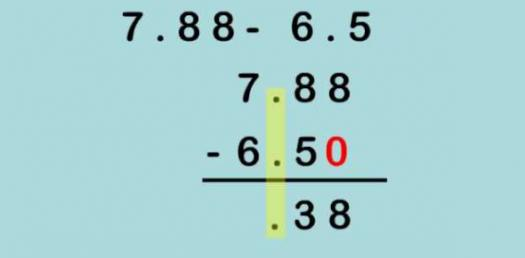14 Questions | Attempts: 1274SettingsThis is 5th Grade Math Test on Adding and Subtracting Decimals. There are a lot of students who have a hard time when it comes to math problems involving decimals and in order to help them out, I have prepared the quiz below covering to functions. Why don’t you give it a try and get to see how well you will do?

• 1.
Please place these decimals in order from LEAST to GREATEST. 8.102 7.019 7.35 6.048 5.81 Write them in the box below:
• 2.
In the number 4.391, what place is the 9 in?
• A.

Tenths

• B.

Ones

• C.

Thousandths

• D.

Hundredths

• 3.
Round  4.18  to the nearest TENTH
• A.

4.20

• B.

4.10

• C.

4.8

• 4.
What is the expanded form of:    82.64
• A.

800 + 20 + .06 + .004

• B.

80 + 2 + .6 + .04

• C.

8 + 2 + .6 + .004

• 5.
What is the expanded form of:  13.274
• A.

(3 x 10) + (2 x .1) + (7 x .1) + (4 x .001)

• B.

(3 x 1) + (2 x 10) + (4 x .1) + (7 x .001)

• C.

(1 x 10) + (3 x 1) + (2 x .1) + (7 x .01) + (4 x .001)

• 6.
What does this hundredths grid represent?
• A.

0.33

• B.

0.28

• C.

0.08

• 7.
Which ordered pair is represented by point X?
• A.

(4, 2)

• B.

(1, 5)

• C.

(2, 4)

• 8.
Which ordered pair is represented by point Z?
• A.

(3, 2)

• B.

(1, 5)

• C.

(2, 4)

• 9.
Round 6.184  to the nearest WHOLE
• A.

7.1

• B.

7

• C.

6

• 10.
Estimate the answer for this problem: 13.6  +  19.82 = ?
• A.

12 + 19 = 31

• B.

14 + 20 = 34

• C.

14 + 18 = 32

• 11.
Solve this problem:  (use a piece of paper to line up decimals and add) 13.6  +  19.82
• A.

33.42

• B.

34.12

• C.

31.38

• 12.
Estimate the answer for this problem: 65.22 - 4.715  = ?
• A.

69 - 3 = 66

• B.

64 - 3 = 61

• C.

65 - 5 = 60

• 13.
Solve this problem:  (Use a piece of paper to line up decimals and subtract) 65.22 - 4.715  = ?
• A.

60.505

• B.

62.350

• C.

61.402

## Related TopicsBack to top
×

Wait!
Here's an interesting quiz for you.